# Electric field due to two charges

• ChiralSuperfields
In summary, @Callumnc1's expression for the electric field due to a point charge at another arbitrary point is not a unit vector. He needs to normalize it so that the direction of the vector is specified.

#### ChiralSuperfields

Homework Statement
Relevant Equations
The problem and solution is,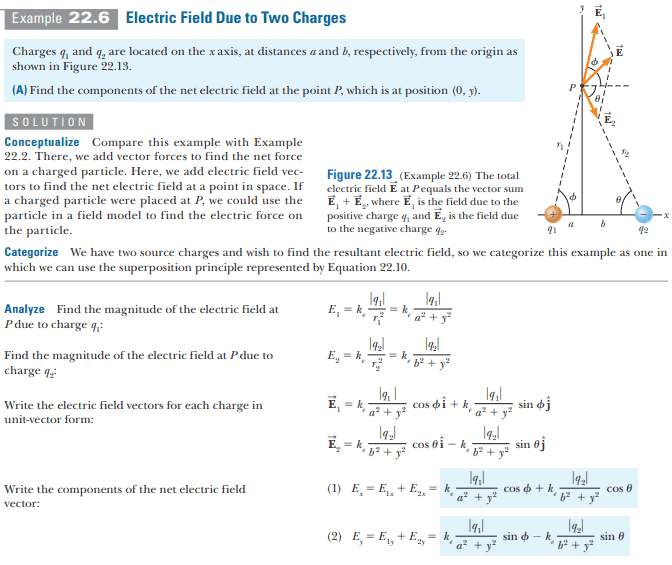However, I would like to know why it cannot be solved like this: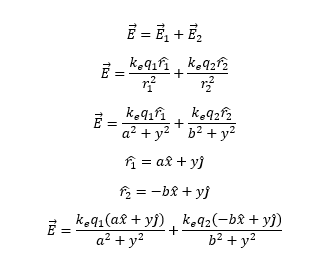Thanks for any help!

•MatinSAR
Callumnc1 said:
View attachment 320262
Thanks for any help!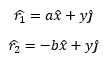Are these unit vectors?

•MatinSAR and ChiralSuperfields
TSny said:
View attachment 320263
Are these unit vectors?
Thanks for your reply @TSny! Yes the two r hat vectors are unit vectors. I guess then they should both have a magnitude of 1. How would you write the x hat and y hat unit vectors in terms of the r hat vectors?

Last edited:
•MatinSAR
Your expression is dimensionally incorrect. The spatial dependence goes as the inverse length not as the inverse length squared. As @TSny noted, you need unit vectors.

If you have a point charge ##q## at position ##\mathbf{r'}##, the electric field vector at a point located at ##\mathbf{r}## is given by $$\mathbf{E}=\frac{k_e~ q(\mathbf{r}-\mathbf{r'})}{|\mathbf{r}-\mathbf{r'}|^3}.$$ To see how it works in this case, consider the negative charge ##q_2##.
Its position is ##~~\mathbf{r'}_2=b~\hat i##
The point of interest is at ##~~\mathbf{r}=y~\hat j##
Then
##\mathbf{r}-\mathbf{r'}_2=-b~\hat i+y~\hat j~;~~## ##|\mathbf{r}-\mathbf{r'}_2|=(b^2+y^2)^{1/2}##
With this mind, the electric field due to ##q_2## is $$\mathbf{E_2}=\frac{k_e ~q_2(-b~\hat i+y~\hat j)}{ \left(b^2+y^2\right)^{3/2}}.$$ Note that this expression is a magnitude $$E_2=\frac{k_e ~q_2}{ b^2+y^2}$$ multiplied by a unit vector $$\mathbf{\hat e}_2=\frac{(-b~\hat i+y~\hat j)}{ \left(b^2+y^2\right)^{1/2}}.$$Your expression was almost there.

•MatinSAR and ChiralSuperfields
kuruman said:
Your expression is dimensionally incorrect. The spatial dependence goes as the inverse length not as the inverse length squared. As @TSny noted, you need unit vectors.

If you have a point charge ##q## at position ##\mathbf{r'}##, the electric field vector at a point located at ##\mathbf{r}## is given by $$\mathbf{E}=\frac{k_e~ q(\mathbf{r}-\mathbf{r'})}{|\mathbf{r}-\mathbf{r'}|^3}.$$ To see how it works in this case, consider the negative charge ##q_2##.
Its position is ##~~\mathbf{r'}_2=b~\hat i##
The point of interest is at ##~~\mathbf{r}=y~\hat j##
Then
##\mathbf{r}-\mathbf{r'}_2=-b~\hat i+y~\hat j~;~~## ##|\mathbf{r}-\mathbf{r'}_2|=(b^2+y^2)^{1/2}##
With this mind, the electric field due to ##q_2## is $$\mathbf{E_2}=\frac{k_e ~q_2(-b~\hat i+y~\hat j)}{ \left(b^2+y^2\right)^{3/2}}.$$ Note that this expression is a magnitude $$E_2=\frac{k_e ~q_2}{ b^2+y^2}$$ multiplied by a unit vector $$\mathbf{\hat e}_2=\frac{(-b~\hat i+y~\hat j)}{ \left(b^2+y^2\right)^{1/2}}.$$Your expression was almost there.
Thanks for your reply @kuruman ! Sorry where did you get this expression from?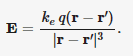I think I did see it once it Kevin Zhou's handouts but I have not really formally learnt it.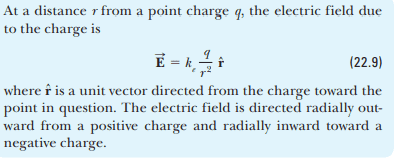I see by inspection that r hat vector is equal to r^ = r - r'. However, why is the absolute value of r - r' cubed?

Many thanks!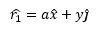The magnitude of the right-hand side is ## |a \hat x + y \hat j| = \sqrt{a^2+y^2}##. This is not equal to 1, so ##a \hat x + y \hat j## is not a unit vector.

But we can get a unit vector representing ##\hat r_1## by dividing ##a \hat x + y \hat j## by ##\sqrt{a^2+y^2}##. Thus, $$\hat r_1 = \frac{a \hat x + y \hat j}{\sqrt{a^2+y^2}}$$ Now, consider the x-component of ##\hat r_1##: $$\frac{a }{\sqrt{a^2+y^2}}$$ How is this related to the angle ##\phi##?

•MatinSAR and ChiralSuperfields
kuruman said:
The spatial dependence goes as the inverse length not as the inverse length squared.
Did you mean that the other way around?
It seems @Callumnc1 intended ##\hat r_1## etc. as unit vectors, but failed to normalise to ensure that they are.

•MatinSAR and ChiralSuperfields
haruspex said:
Did you mean that the other way around?
I meant that the spatial dependence of @Callumnc1's goes as the inverse length when it ought to go as the inverse length squared. Yes, the expression has the right direction but was not properly normalized. That is why I said that his expression was "almost there."

•MatinSAR and ChiralSuperfields
Callumnc1 said:
Thanks for your reply @kuruman ! Sorry where did you get this expression from?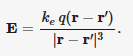It's the most general expression for the electric field at an arbitrary point due to a point charge placed at another arbitrary point. It can be found in any intermediate-level E&M textbook and on the web.
Callumnc1 said:
think I did see it once it Kevin Zhou's handouts but I have not really formally learnt it.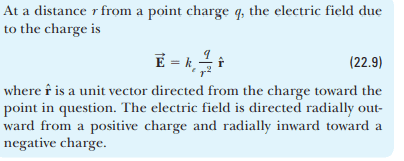Perhaps now is the time to learn it. The one you know, equation (22.9), is not general enough because it assumes that the point charge is located at the origin. How would you express the electric field vector if the origin has already been chosen for you and the charge is not at it?

Callumnc1 said:
I see by inspection that r hat vector is equal to r^ = r - r'.
That equation has problems
1. It is not a vector equation
2. The right hand side is not a unit vector.

Question: How would you write a unit vector in the direction of an arbitrary vector?
Answer: You divide the vector by its magnitude. See posts #4 and #6.

•MatinSAR and ChiralSuperfields
TSny said:
The magnitude of the right-hand side is ## |a \hat x + y \hat j| = \sqrt{a^2+y^2}##. This is not equal to 1, so ##a \hat x + y \hat j## is not a unit vector.

But we can get a unit vector representing ##\hat r_1## by dividing ##a \hat x + y \hat j## by ##\sqrt{a^2+y^2}##. Thus, $$\hat r_1 = \frac{a \hat x + y \hat j}{\sqrt{a^2+y^2}}$$ Now, consider the x-component of ##\hat r_1##: $$\frac{a }{\sqrt{a^2+y^2}}$$ How is this related to the angle ##\phi##?
Thanks for your replies @TSny, I think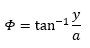. But since you said that the x-component of the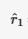was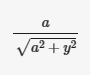then a =so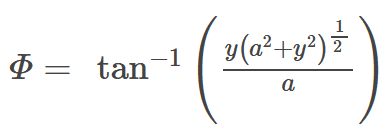But to be honest, it dose seem a bit strange that a =, did I do something wrong?

Many thanks!

•MatinSAR
haruspex said:
Did you mean that the other way around?
It seems @Callumnc1 intended ##\hat r_1## etc. as unit vectors, but failed to normalise to ensure that they are.
Thank you @haruspex!

•MatinSAR
You are complicating things unnecessarily. Look at the ratio ##\dfrac{a }{\sqrt{a^2+y^2}}##. Now look at the drawing. The denominator is the hypotenuse of the right triangle on the left with ##q _1## at its corner. Right side ##a## is adjacent to given angle ##\phi##. So the ratio is $$\rm{Ratio}=\frac{\rm{Adjacent}}{\rm{Hypotenuse}}.$$ Does this ring a bell?

•MatinSAR and ChiralSuperfields
kuruman said:
It's the most general expression for the electric field at an arbitrary point due to a point charge placed at another arbitrary point. It can be found in any intermediate-level E&M textbook and on the web.

Perhaps now is the time to learn it. The one you know, equation (22.9), is not general enough because it assumes that the point charge is located at the origin. How would you express the electric field vector if the origin has already been chosen for you and the charge is not at it?

That equation has problems
1. It is not a vector equation
2. The right hand side is not a unit vector.

Question: How would you write a unit vector in the direction of an arbitrary vector?
Answer: You divide the vector by its magnitude. See posts #4 and #6.
Thank you for your help @kuruman ! That is a useful expression you mentioned! So I see that the equation 22.9 that I mentioned is a special case of your equation when the r' = 0 when the charge is at the origin. Then the equation simplifies to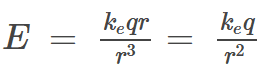, however, where do they get the unit vector from that is shown in equation 22.9?

Also how is my equation not a vector equation since r hat is a unit vector and both r and r' are position vectors?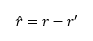Many thanks!

Callumnc1 said:
I think ##\phi = \tan^{-1} \frac y a##.
Yes, this is correct. Can you express ##\frac a {\sqrt{a^2 + y^2}}## as some trig function of ##\phi##?
Callumnc1 said:
But to be honest, it dose seem a bit strange that ## a = \frac a {\sqrt{a^2+y^2}}## , did I do something wrong?
Right, this equation is not correct. I don’t see how you arrived at it. Remember, your original equation ##\hat r_1 =a \hat i+ y \hat j## for the unit vector ##\hat r_1## is not correct. So the x-component of ##\hat r_1## is not equal to ##a##.

•MatinSAR and ChiralSuperfields
kuruman said:
I meant that the spatial dependence of @Callumnc1's goes as the inverse length when it ought to go as the inverse length squared. Yes, the expression has the right direction but was not properly normalized. That is why I said that his expression was "almost there."
Ah, ok - it was just ambiguous as to which was which in post #4.

•MatinSAR and ChiralSuperfields
TSny said:
Yes, this is correct. Can you express ##\frac a {\sqrt{a^2 + y^2}}## as some trig function of ##\phi##?

Right, this equation is not correct. I don’t see how you arrived at it. Remember, your original equation ##\hat r_1 =a \hat i+ y \hat j## for the unit vector ##\hat r_1## is not correct. So the x-component of ##\hat r_1## is not equal to ##a##.
Thank you @TSny and @kuruman for your replies! So I assume that the only way to find the x-component of the unit vector is to set y = 0 (let me know if you guys know any other ways), therefore the x-component is of the r hat unit vector is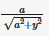. I now think that the trig function can be expressed as cos (phi) =.

Many thanks!

kuruman said:
You are complicating things unnecessarily. Look at the ratio ##\dfrac{a }{\sqrt{a^2+y^2}}##. Now look at the drawing. The denominator is the hypotenuse of the right triangle on the left with ##q _1## at its corner. Right side ##a## is adjacent to given angle ##\phi##. So the ratio is $$\rm{Ratio}=\frac{\rm{Adjacent}}{\rm{Hypotenuse}}.$$ Does this ring a bell?
Yes sorry @kuruman , I understand now. :)